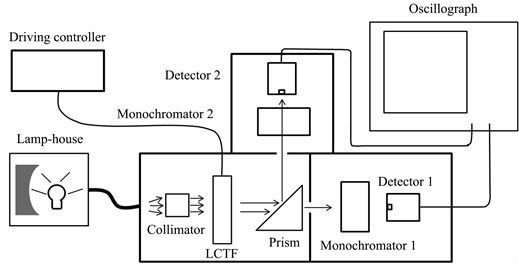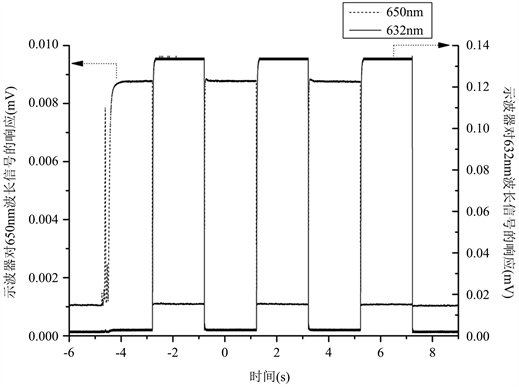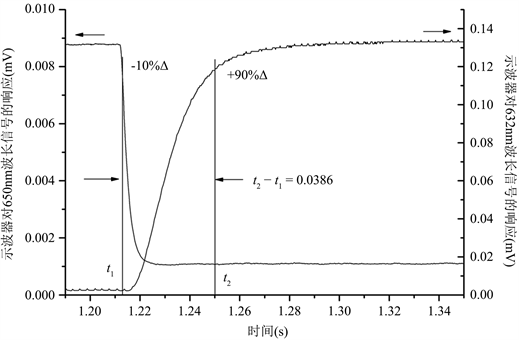﻿ Lyot型液晶可调滤光片波长切换响应时间的精确测量

# Lyot型液晶可调滤光片波长切换响应时间的精确测量Precision Measurement of Wavelength Switch Response Time for Liquid Crystal Tunable Filter

Abstract: Lyot-type liquid crystal tunable filters (LCTF) are widely used as fast switch filters. A general value or a range of the response time of wavelength switch given in the product manual can’t support the efficient uses of instruments when the response time should be controlled rigorously. This paper presents a precise method of measuring the response time of wavelength switch for Lyot-type LCTF. Simultaneous measurement of the light intensity of the starting wavelength and ending wavelength is proposed, and the result is obtained by the analysis of the recorded data. The feasibility of this method is validated by the experiment of a visible light LCTF, and the fine precision is presented by the analysis of measurement uncertainty.

1. 引言

2. 测量原理与方法

${T}_{1}=\frac{1}{2}\left(1+\mathrm{cos}{\delta }_{1}\right)=\frac{1}{2}\left(1+\mathrm{cos}\frac{2\pi \Delta {n}_{1}{d}_{1}}{\lambda }\right)$ (1)

${\delta }_{1}$ 为o光和e光在第1级产生的相位差， $\Delta {n}_{1}$ 为o光和e光的在第1级液晶层中的折射率差， ${d}_{1}$ 为第1级液晶层的厚度，λ为光的波长。

${T}_{i}=\frac{1}{2}\left(1+\mathrm{cos}{\delta }_{i}\right)$ (2)

$T={\prod }_{i=1}^{m}{T}_{i}$ (3)Figure 1. LCTF wavelength switch response time measurement device

3. 实验及结果Figure 2. Figure of Wavelength switch signal collected by oscilloscopeFigure 3. Example for 650 nm switch to 632 nm data processingTable 1. System resulting data of standard experiment

4. 不确定度分析

1) 由采样率引入的测量不确定度 ${u}_{1}$

$u\left(t\right)=0.1/\sqrt{3}\approx 0.06\text{\hspace{0.17em}}\text{ms}$ (4)

${u}_{1}=\sqrt{{u}^{2}\left({t}_{1}\right)+{u}^{2}\left({t}_{2}\right)}=\sqrt{2}u\left(t\right)\approx 0.1\text{\hspace{0.17em}}\text{ms}$ (5)

2) 由时基精度引入的测量不确定度 ${u}_{2}$

${u}_{2}=0.002/\sqrt{3}\approx 0.001\text{\hspace{0.17em}}\text{ms}$ (6)

3) 由脉冲幅度的不准确测量引入的测量不确定度 ${u}_{3}$

${u}_{3}=\sqrt{{0.04}^{2}+{0.16}^{2}}\approx 0.16\text{\hspace{0.17em}}\text{ms}$ (7)

4) 由测量重复性引入的测量不确定度 ${u}_{4}$

${u}_{4}=\frac{R}{C\sqrt{n}}=\frac{0.5}{1.69×\sqrt{3}}\approx 0.2\text{\hspace{0.17em}}\text{ms}$ (8)

${u}_{c}=\sqrt{{u}_{1}^{2}+{u}_{2}^{2}+{u}_{3}^{2}+{u}_{4}^{2}}=\sqrt{{0.1}^{2}+{0.001}^{2}+{0.16}^{2}+{0.2}^{2}}\approx 0.3\text{\hspace{0.17em}}\text{ms}$ (9)

95%置信区间的扩展测量不确定U = 0.6 ms，以3次测量的平均值表示测量结果时，650 nm切换到632 nm的响应时间是(38.6 ± 0.6) ms；k = 2。

5. 结论

NOTES

*通讯作者。

 曹星烨. 基于液晶可调谐的宽光谱窄带Lyot型滤光片[D]: [硕士学位论文]. 杭州: 浙江大学, 2008.

 杨国伟, 郑臻荣, 李海峰, 刘旭. Solc型液晶可调谐滤光片的研究[J]. 光学学报, 2010, 30(6): 1383-1387.

 Liquid Crystal Tunable Filters Data Sheet [EB/OL]. http://www.iseeimaging.com/hardware/CRI/cri.htm, 2020-7-2

 Liquid Crystal Tunable Filter User Manual, Revision 2.00 [EB/OL]. https://www.meadowlark.com/store/D4020_User_Manual_2.0.pdf, 2021-6-10.

 Wang, H.Y. (2005) Studies of Liquid Crystal Response Time. Thesis, University of Central Florida, Orlando.

 Varispec Liquid Crystal Tunable Filters User’s Manual, Revision D [EB/OL]. https://www.perkinelmer.com.cn/CMSResources/Images/46-140159MAN_LST_Q112_VarispecUserManual1107-9252.pdf, 2020-6-10.

 Selectable Bandwidth Tunable Optical Filter [EB/OL]. https://www.meadowlark.com/store/data_sheet/LC-Selectable_Bandwidth_Tunable_Optical_Filter.pdf, 2021-6-10.

 Yozo, U., Toshihisa, K., Ryo, N. and Katsuhiko, S. (2005) Measurement Methods of Nematic Liquid Crystal Response Time. Molecular Crystals and Liquid Crystals, 434, 337-352.
https://doi.org/10.1080/15421400590954452

 李剑, 王海燕, 李强, 等. SJ/T11348-2006数字电视平板显示器测量方法[S]. 北京: 中华人民共和国信息产业部, 2006.

 Wu, S.T. (1989) Design of a Liquid Crystal Based Tunable Electrooptic Filter. Applied Optics, 28, 48-52.
https://doi.org/10.1364/AO.28.000048

Top